# Paint graphical objectsBrowse the sample

.NET Multi-platform App UI (.NET MAUI) graphics includes the ability to paint graphical objects with solid colors, gradients, repeating images, and patterns.

The Paint class is an abstract class that paints an object with its output. Classes that derive from Paint describe different ways of painting an object. The following list describes the different paint types available in .NET MAUI graphics:

Instances of these types can be painted on an ICanvas, typically by using the SetFillPaint method to set the paint as the fill of a graphical object.

The Paint class also defines BackgroundColor, and ForegroundColor properties, of type Color, that can be used to optionally define background and foreground colors for a Paint object.

## Paint a solid color

The SolidPaint class, that's derived from the Paint class, is used to paint a graphical object with a solid color.

The SolidPaint class defines a Color property, of type Color, which represents the color of the paint. The class also has an IsTransparent property that returns a `bool` that represents whether the color has an alpha value of less than 1.

### Create a SolidPaint object

The color of a SolidPaint object is typically specified through its constructor, using a Color argument:

``````SolidPaint solidPaint = new SolidPaint(Colors.Silver);

RectF solidRectangle = new RectF(100, 100, 200, 100);
canvas.SetFillPaint(solidPaint, solidRectangle);
canvas.FillRoundedRectangle(solidRectangle, 12);
``````

The SolidPaint object is specified as the first argument to the SetFillPaint method. Therefore, a filled rounded rectangle is painted with a silver SolidPaint object: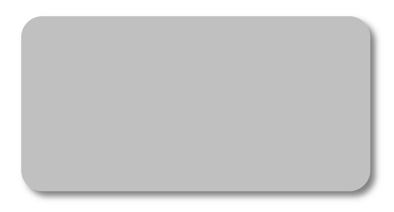Alternatively, the color can be specified with the Color property:

``````SolidPaint solidPaint = new SolidPaint
{
Color = Colors.Silver
};

RectF solidRectangle = new RectF(100, 100, 200, 100);
canvas.SetFillPaint(solidPaint, solidRectangle);
canvas.FillRoundedRectangle(solidRectangle, 12);
``````

## Paint an image

The ImagePaint class, that's derived from the Paint class, is used to paint a graphical object with an image.

The ImagePaint class defines an Image property, of type IImage, which represents the image to paint. The class also has an IsTransparent property that returns `false`.

### Create an ImagePaint object

To paint an object with an image, load the image and assign it to the Image property of the ImagePaint object.

Note

Loading an image that's embedded in an assembly requires the image to have its build action set to Embedded Resource.

The following example shows how to load an image and fill a rectangle with it:

``````using Microsoft.Maui.Graphics.Platform;
...

IImage image;
var assembly = GetType().GetTypeInfo().Assembly;
using (var stream = assembly.GetManifestResourceStream("GraphicsViewDemos.Resources.Images.dotnet_bot.png"))
{
image = PlatformImage.FromStream(stream);
}

if (image != null)
{
ImagePaint imagePaint = new ImagePaint
{
Image = image.Downsize(100)
};
canvas.SetFillPaint(imagePaint, RectF.Zero);
canvas.FillRectangle(0, 0, 240, 300);
}
``````

Warning

The PlatformImage type isn't supported on Windows.

In this example, the image is retrieved from the assembly and loaded as a stream. The image is resized using the Downsize method, with the argument specifying that its largest dimension should be set to 100 pixels. For more information about downsizing an image, see Downsize an image.

The Image property of the ImagePaint object is set to the downsized version of the image, and the ImagePaint object is set as the paint to fill an object with. A rectangle is then drawn that's filled with the paint: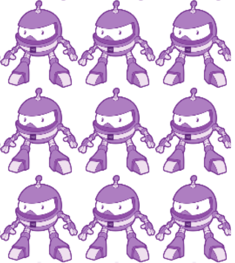Note

An ImagePaint object can also be created from an IImage object by the `AsPaint` extension method.

Alternatively, the SetFillImage extension method can be used to simplify the code:

``````if (image != null)
{
canvas.SetFillImage(image.Downsize(100));
canvas.FillRectangle(0, 0, 240, 300);
}
``````

## Paint a pattern

The PatternPaint class, that's derived from the Paint class, is used to paint a graphical object with a pattern.

The PatternPaint class defines a Pattern property, of type IPattern, which represents the pattern to paint. The class also has an IsTransparent property that returns a `bool` that represents whether the background or foreground color of the paint has an alpha value of less than 1.

### Create a PatternPaint object

To paint an area with a pattern, create the pattern and assign it to the Pattern property of a PatternPaint object.

The following example shows how to create a pattern and fill an object with it:

``````IPattern pattern;

// Create a 10x10 template for the pattern
using (PictureCanvas picture = new PictureCanvas(0, 0, 10, 10))
{
picture.StrokeColor = Colors.Silver;
picture.DrawLine(0, 0, 10, 10);
picture.DrawLine(0, 10, 10, 0);
pattern = new PicturePattern(picture.Picture, 10, 10);
}

// Fill the rectangle with the 10x10 pattern
PatternPaint patternPaint = new PatternPaint
{
Pattern = pattern
};
canvas.SetFillPaint(patternPaint, RectF.Zero);
canvas.FillRectangle(10, 10, 250, 250);
``````

In this example, the pattern is a 10x10 area that contains a diagonal line from (0,0) to (10,10), and a diagonal line from (0,10) to (10,0). The Pattern property of the PatternPaint object is set to the pattern, and the PatternPaint object is set as the paint to fill an object with. A rectangle is then drawn that's filled with the paint:Note

A PatternPaint object can also be created from a `PicturePattern` object by the `AsPaint` extension method.

The GradientPaint class, that's derived from the Paint class, is an abstract base class that describes a gradient, which is composed of gradient steps. A GradientPaint paints a graphical object with multiple colors that blend into each other along an axis. Classes that derive from GradientPaint describe different ways of interpreting gradients stops, and .NET MAUI graphics provides the following gradient paints:

The GradientPaint class defines the GradientStops property, of type PaintGradientStop, which represents the brush's gradient stops, each of which specifies a color and an offset along the gradient axis.

The PaintGradientStop class defines the following properties:

• Color, of type Color, which represents the color of the gradient stop.
• Offset, of type `float`, which represents the location of the gradient stop within the gradient vector. Valid values are in the range 0.0-1.0. The closer this value is to 0, the closer the color is to the start of the gradient. Similarly, the closer this value is to 1, the closer the color is to the end of the gradient.

Important

The coordinate system used by gradients is relative to a bounding box for the graphical object. 0 indicates 0 percent of the bounding box, and 1 indicates 100 percent of the bounding box. Therefore, (0.5,0.5) describes a point in the middle of the bounding box, and (1,1) describes a point at the bottom right of the bounding box.

The following example creates a diagonal LinearGradientPaint with four colors:

``````LinearGradientPaint linearGradientPaint = new LinearGradientPaint
{
StartColor = Colors.Yellow,
EndColor = Colors.Green,
StartPoint = new Point(0, 0),
EndPoint = new Point(1, 1)
};

RectF linearRectangle = new RectF(10, 10, 200, 100);
canvas.FillRoundedRectangle(linearRectangle, 12);
``````

The color of each point between gradient stops is interpolated as a combination of the color specified by the two bounding gradient stops. The following diagram shows the gradient stops from the previous example: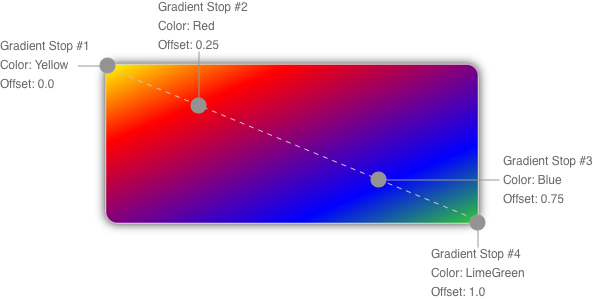The LinearGradientPaint class defines the following properties:

• StartPoint, of type Point, which represents the starting two-dimensional coordinates of the linear gradient. The class constructor initializes this property to (0,0).
• EndPoint, of type Point, which represents the ending two-dimensional coordinates of the linear gradient. The class constructor initializes this property to (1,1).

A linear gradient's gradient stops are positioned along the gradient axis. The orientation and size of the gradient axis can be changed using the StartPoint and EndPoint properties. By manipulating these properties, you can create horizontal, vertical, and diagonal gradients, reverse the gradient direction, condense the gradient spread, and more.

The StartPoint and EndPoint properties are relative to the graphical object being painted. (0,0) represents the top-left corner of the object being painted, and (1,1) represents the bottom-right corner of the object being painted. The following diagram shows the gradient axis for a diagonal linear gradient brush:In this diagram, the dashed line shows the gradient axis, which highlights the interpolation path of the gradient from the start point to the end point.

#### Create a horizontal linear gradient

To create a horizontal linear gradient, create a LinearGradientPaint object and set its StartColor and EndColor properties. Then, set its EndPoint to (1,0).

The following example shows how to create a horizontal LinearGradientPaint:

``````LinearGradientPaint linearGradientPaint = new LinearGradientPaint
{
StartColor = Colors.Yellow,
EndColor = Colors.Green,
EndPoint = new Point(1, 0)
};

RectF linearRectangle = new RectF(10, 10, 200, 100);
canvas.FillRoundedRectangle(linearRectangle, 12);
``````

In this example, the rounded rectangle is painted with a linear gradient that interpolates horizontally from yellow to green:#### Create a vertical linear gradient

To create a vertical linear gradient, create a LinearGradientPaint object and set its StartColor and EndColor properties. Then, set its EndPoint to (0,1).

The following example shows how to create a vertical LinearGradientPaint:

``````LinearGradientPaint linearGradientPaint = new LinearGradientPaint
{
StartColor = Colors.Yellow,
EndColor = Colors.Green,
EndPoint = new Point(0, 1)
};

RectF linearRectangle = new RectF(10, 10, 200, 100);
canvas.FillRoundedRectangle(linearRectangle, 12);
``````

In this example, the rounded rectangle is painted with a linear gradient that interpolates vertically from yellow to green: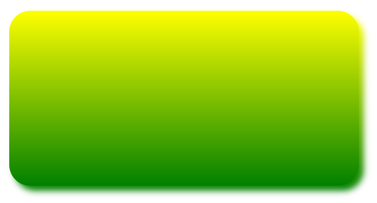#### Create a diagonal linear gradient

To create a diagonal linear gradient, create a LinearGradientPaint object and set its StartColor and EndColor properties.

The following example shows how to create a diagonal LinearGradientPaint:

``````LinearGradientPaint linearGradientPaint = new LinearGradientPaint
{
StartColor = Colors.Yellow,
EndColor = Colors.Green,
};

RectF linearRectangle = new RectF(10, 10, 200, 100);
canvas.FillRoundedRectangle(linearRectangle, 12);
``````

In this example, the rounded rectangle is painted with a linear gradient that interpolates diagonally from yellow to green: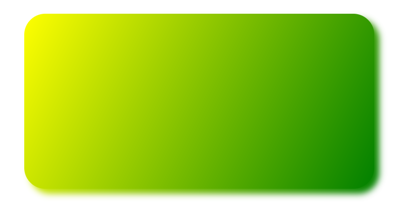• Center, of type Point, which represents the center point of the circle for the radial gradient. The class constructor initializes this property to (0.5,0.5).
• Radius, of type `double`, which represents the radius of the circle for the radial gradient. The class constructor initializes this property to 0.5.

A radial gradient's gradient stops are positioned along a gradient axis defined by a circle. The gradient axis radiates from the center of the circle to its circumference. The position and size of the circle can be changed using the Center and Radius properties. The circle defines the end point of the gradient. Therefore, a gradient stop at 1.0 defines the color at the circle's circumference. A gradient stop at 0.0 defines the color at the center of the circle.

``````RadialGradientPaint radialGradientPaint = new RadialGradientPaint
{
StartColor = Colors.Red,
EndColor = Colors.DarkBlue
};

RectF radialRectangle = new RectF(10, 10, 200, 100);
``````

In this example, the rounded rectangle is painted with a radial gradient that interpolates from red to dark blue. The center of the radial gradient is positioned in the center of the rectangle: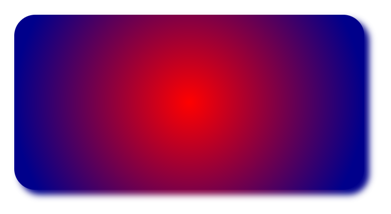The following example moves the center of the radial gradient to the top-left corner of the rectangle:

``````RadialGradientPaint radialGradientPaint = new RadialGradientPaint
{
StartColor = Colors.Red,
EndColor = Colors.DarkBlue,
Center = new Point(0.0, 0.0)
};

RectF radialRectangle = new RectF(10, 10, 200, 100);
``````

In this example, the rounded rectangle is painted with a radial gradient that interpolates from red to dark blue. The center of the radial gradient is positioned in the top-left of the rectangle: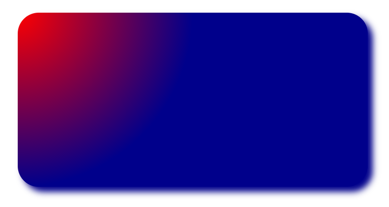The following example moves the center of the radial gradient to the bottom-right corner of the rectangle:

``````RadialGradientPaint radialGradientPaint = new RadialGradientPaint
{
StartColor = Colors.Red,
EndColor = Colors.DarkBlue,
Center = new Point(1.0, 1.0)